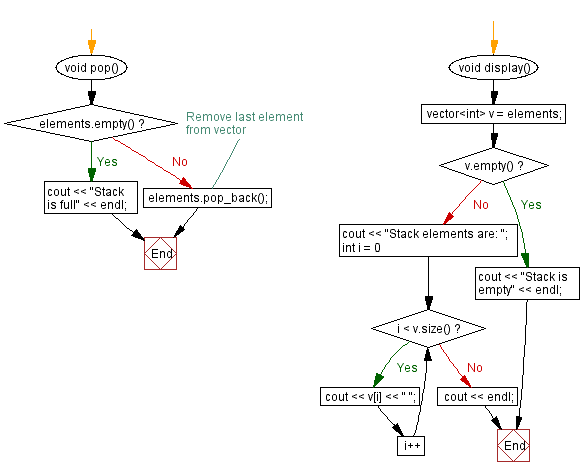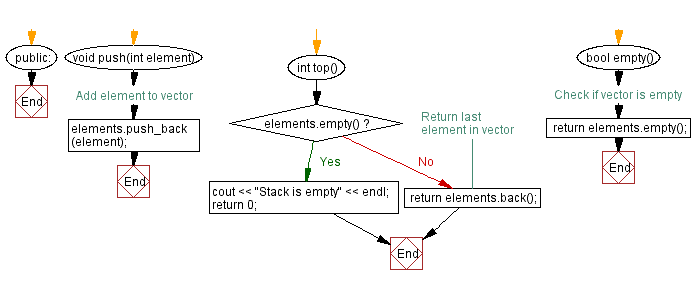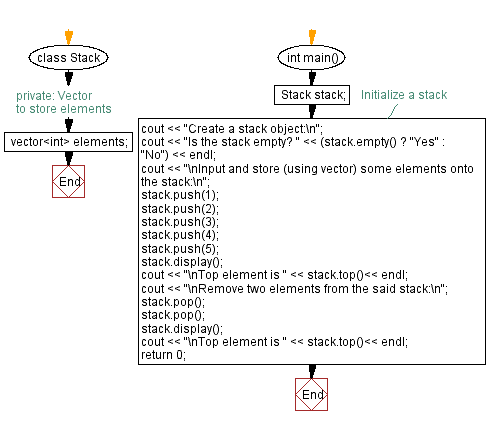﻿ C++ Implement a stack using a vector with push, pop operations

# C++ Stack Exercises: Implement a stack using a vector with push, pop operations

## C++ Stack: Exercise-24 with Solution

Write a C++ program to implement a stack using a vector with push and pop operations. Check if the stack is empty or not and find the top element of the stack.

Test Data:
Create a stack object:
Is the stack empty? Yes
Input and store (using vector) some elements onto the stack:
Stack elements are: 1 2 3 4 5
Top element is 5

Sample Solution:

C++ Code:

``````#include <iostream>

#include <vector>
using namespace std;
class Stack {
private:
// Vector to store elements
vector<int> elements;
public:
void push(int element) {
elements.push_back(element);
}
void pop() {
if (elements.empty()) {
cout << "Stack is full" << endl;
} else {
// Remove last element from vector
elements.pop_back();
}
}
int top() {
if (elements.empty()) {
cout << "Stack is empty" << endl;
return 0;
} else {
// Return last element in vector
return elements.back();
}
}

bool empty() {
// Check if vector is empty
return elements.empty();
}

void display() {
vector<int> v = elements;
if (v.empty()) {
cout << "Stack is empty" << endl;
return;
}
cout << "Stack elements are: ";
for (int i = 0; i < v.size(); i++) {
cout << v[i] << " ";
}
cout << endl;
}
};

int main() {
Stack stack;
//Initialize a stack
cout << "Create a stack object:\n";
cout << "Is the stack empty? " << (stack.empty() ? "Yes" : "No") << endl;
cout << "\nInput and store (using vector) some elements onto the stack:\n";
stack.push(1);
stack.push(2);
stack.push(3);
stack.push(4);
stack.push(5);
stack.display();
cout << "\nTop element is " << stack.top()<< endl;
cout << "\nRemove two elements from the said stack:\n";
stack.pop();
stack.pop();
stack.display();
cout << "\nTop element is " << stack.top()<< endl;
return 0;
}
``````

Sample Output:

```Create a stack object:
Is the stack empty? Yes

Input and store (using vector) some elements onto the stack:
Stack elements are: 1 2 3 4 5

Top element is 5

Remove two elements from the said stack:
Stack elements are: 1 2 3

Top element is 3
```

Flowchart:CPP Code Editor: